Want to share your content on R-bloggers? click here if you have a blog, or here if you don't.

In genomic fields, it’s very common to explore the gene expression profile of one or a list of genes involved in a pathway of interest. Here, we present some helper functions in the ggpubr R package to facilitate exploratory data analysis (EDA) for life scientists.Exploratory Data visualization: Gene Expression Data

Standard graphical techniques used in EDA, include:

• Box plot
• Violin plot
• Stripchart
• Dot plot
• Histogram and density plots
• ECDF plot
• Q-Q plot

All these plots can be created using the ggplot2 R package, which is highly flexible.

However, to customize a ggplot, the syntax might appear opaque for a beginner and this raises the level of difficulty for researchers with no advanced R programming skills. If you’re not familiar with ggplot2 system, you can start by reading our Guide to Create Beautiful Graphics in R.

Previously, we described how to Add P-values and Significance Levels to ggplots. In this article, we present the ggpubr package, a wrapper around ggplot2, which provides some easy-to-use functions for creating ‘ggplot2’- based publication ready plots. We’ll use the ggpubr functions to visualize gene expression profile from TCGA genomic data sets.

Contents:

## Prerequisites

### ggpubr package

Required R package: ggpubr (version >= 0.1.3).

• Install from CRAN as follow:

`install.packages("ggpubr")`

• Or, install the latest developmental version from GitHub as follow:

```if(!require(devtools)) install.packages("devtools")
devtools::install_github("kassambara/ggpubr")```

`library(ggpubr)`

### TCGA data

The Cancer Genome Atlas (TCGA) data is a publicly available data containing clinical and genomic data across 33 cancer types. These data include gene expression, CNV profiling, SNP genotyping, DNA methylation, miRNA profiling, exome sequencing, and other types of data.

The RTCGA R package, by Marcin Marcin Kosinski et al., provides a convenient solution to access to clinical and genomic data available in TCGA. Each of the data packages is a separate package, and must be installed (once) individually.

The following R code installs the core RTCGA package as well as the clinical and mRNA gene expression data packages.

```# Load the bioconductor installer.
source("https://bioconductor.org/biocLite.R")

# Install the main RTCGA package
biocLite("RTCGA")

# Install the clinical and mRNA gene expression data packages
biocLite("RTCGA.clinical")
biocLite("RTCGA.mRNA")```

To see the type of data available for each cancer type, use this:

```library(RTCGA)
infoTCGA()```

```# A tibble: 38 x 13
Cohort    BCR Clinical     CN   LowP Methylation   mRNA mRNASeq    miR miRSeq   RPPA    MAF rawMAF
*   <fctr> <fctr>   <fctr> <fctr> <fctr>      <fctr> <fctr>  <fctr> <fctr> <fctr> <fctr> <fctr> <fctr>
1      ACC     92       92     90      0          80      0      79      0     80     46     90      0
2     BLCA    412      412    410    112         412      0     408      0    409    344    130    395
3     BRCA   1098     1097   1089     19        1097    526    1093      0   1078    887    977      0
4     CESC    307      307    295     50         307      0     304      0    307    173    194      0
5     CHOL     51       45     36      0          36      0      36      0     36     30     35      0
6     COAD    460      458    451     69         457    153     457      0    406    360    154    367
7 COADREAD    631      629    616    104         622    222     623      0    549    491    223    489
8     DLBC     58       48     48      0          48      0      48      0     47     33     48      0
9     ESCA    185      185    184     51         185      0     184      0    184    126    185      0
10     FPPP     38       38      0      0           0      0       0      0     23      0      0      0
# ... with 28 more rows```

## Gene expression data

The R function expressionsTCGA() [in RTCGA package] can be used to easily extract the expression values of genes of interest in one or multiple cancer types.

In the following R code, we start by extracting the mRNA expression for five genes of interest – GATA3, PTEN, XBP1, ESR1 and MUC1 – from 3 different data sets:

• Breast invasive carcinoma (BRCA),
• Ovarian serous cystadenocarcinoma (OV) and
• Lung squamous cell carcinoma (LUSC)

```library(RTCGA)
library(RTCGA.mRNA)
expr <- expressionsTCGA(BRCA.mRNA, OV.mRNA, LUSC.mRNA,
extract.cols = c("GATA3", "PTEN", "XBP1","ESR1", "MUC1"))
expr```

```# A tibble: 1,305 x 7
bcr_patient_barcode   dataset     GATA3       PTEN      XBP1       ESR1      MUC1
<chr>     <chr>     <dbl>      <dbl>     <dbl>      <dbl>     <dbl>
1 TCGA-A1-A0SD-01A-11R-A115-07 BRCA.mRNA  2.870500  1.3613571  2.983333  3.0842500  1.652125
2 TCGA-A1-A0SE-01A-11R-A084-07 BRCA.mRNA  2.166250  0.4283571  2.550833  2.3860000  3.080250
3 TCGA-A1-A0SH-01A-11R-A084-07 BRCA.mRNA  1.323500  1.3056429  3.020417  0.7912500  2.985250
4 TCGA-A1-A0SJ-01A-11R-A084-07 BRCA.mRNA  1.841625  0.8096429  3.131333  2.4954167 -1.918500
5 TCGA-A1-A0SK-01A-12R-A084-07 BRCA.mRNA -6.025250  0.2508571 -1.451750 -4.8606667 -1.171500
6 TCGA-A1-A0SM-01A-11R-A084-07 BRCA.mRNA  1.804500  1.3107857  4.041083  2.7970000  3.529750
7 TCGA-A1-A0SO-01A-22R-A084-07 BRCA.mRNA -4.879250 -0.2369286 -0.724750 -4.4860833 -1.455750
8 TCGA-A1-A0SP-01A-11R-A084-07 BRCA.mRNA -3.143250 -1.2432143 -1.193083 -1.6274167 -0.986750
9 TCGA-A2-A04N-01A-11R-A115-07 BRCA.mRNA  2.034000  1.2074286  2.278833  4.1155833  0.668000
10 TCGA-A2-A04P-01A-31R-A034-07 BRCA.mRNA -0.293125  0.2883571 -1.605083  0.4731667  0.011500
# ... with 1,295 more rows```

To display the number of sample in each data set, type this:

```nb_samples <- table(expr\$dataset)
nb_samples```

```BRCA.mRNA LUSC.mRNA   OV.mRNA
590       154       561```

We can simplify data set names by removing the “mRNA” tag. This can be done using the R base function gsub().

`expr\$dataset <- gsub(pattern = ".mRNA", replacement = "",  expr\$dataset)`

Let’s simplify also the patients’ barcode column. The following R code will change the barcodes into BRCA1, BRCA2, …, OV1, OV2, …., etc

```expr\$bcr_patient_barcode <- paste0(expr\$dataset, c(1:590, 1:561, 1:154))
expr```

```# A tibble: 1,305 x 7
bcr_patient_barcode dataset     GATA3       PTEN      XBP1       ESR1      MUC1
<chr>   <chr>     <dbl>      <dbl>     <dbl>      <dbl>     <dbl>
1               BRCA1    BRCA  2.870500  1.3613571  2.983333  3.0842500  1.652125
2               BRCA2    BRCA  2.166250  0.4283571  2.550833  2.3860000  3.080250
3               BRCA3    BRCA  1.323500  1.3056429  3.020417  0.7912500  2.985250
4               BRCA4    BRCA  1.841625  0.8096429  3.131333  2.4954167 -1.918500
5               BRCA5    BRCA -6.025250  0.2508571 -1.451750 -4.8606667 -1.171500
6               BRCA6    BRCA  1.804500  1.3107857  4.041083  2.7970000  3.529750
7               BRCA7    BRCA -4.879250 -0.2369286 -0.724750 -4.4860833 -1.455750
8               BRCA8    BRCA -3.143250 -1.2432143 -1.193083 -1.6274167 -0.986750
9               BRCA9    BRCA  2.034000  1.2074286  2.278833  4.1155833  0.668000
10              BRCA10    BRCA -0.293125  0.2883571 -1.605083  0.4731667  0.011500
# ... with 1,295 more rows```

The above (expr) dataset has been saved at https://raw.githubusercontent.com/kassambara/data/master/expr_tcga.txt. This data is required to practice the R code provided in this tutotial.

If you experience some issues in installing the RTCGA packages, You can simply load the data as follow:

```expr <- read.delim("https://raw.githubusercontent.com/kassambara/data/master/expr_tcga.txt",
stringsAsFactors = FALSE)```

## Box plots

Create a box plot of a gene expression profile, colored by groups (here data set/cancer type):

```library(ggpubr)
# GATA3
ggboxplot(expr, x = "dataset", y = "GATA3",
title = "GATA3", ylab = "Expression",
color = "dataset", palette = "jco")

# PTEN
ggboxplot(expr, x = "dataset", y = "PTEN",
title = "PTEN", ylab = "Expression",
color = "dataset", palette = "jco")```Exploratory Data visualization: Gene Expression Data

Note that, the argument palette is used to change color palettes. Allowed values include:

• “grey” for grey color palettes;
• brewer palettes e.g. “RdBu”, “Blues”, …;. To view all, type this in R: RColorBrewer::display.brewer.all() or click here to see all brewer palettes;
• or custom color palettes e.g. c(“blue”, “red”) or c(“#00AFBB”, “#E7B800”);
• and scientific journal palettes from the ggsci R package, e.g.: “npg”, “aaas”, “lancet”, “jco”, “ucscgb”, “uchicago”, “simpsons” and “rickandmorty”.

Instead of repeating the same R code for each gene, you can create a list of plots at once, as follow:

```# Create a  list of plots
p <- ggboxplot(expr, x = "dataset",
y = c("GATA3", "PTEN", "XBP1"),
title = c("GATA3", "PTEN", "XBP1"),
ylab = "Expression",
color = "dataset", palette = "jco")

# View GATA3
p\$GATA3

# View PTEN
p\$PTEN

# View XBP1
p\$XBP1```

Note that, when the argument y contains multiple variables (here multiple gene names), then the arguments title, xlab and ylab can be also a character vector of same length as y.

To add p-values and significance levels to the boxplots, read our previous article: Add P-values and Significance Levels to ggplots. Briefly, you can do this:

```my_comparisons <- list(c("BRCA", "OV"), c("OV", "LUSC"))
ggboxplot(expr, x = "dataset", y = "GATA3",
title = "GATA3", ylab = "Expression",
color = "dataset", palette = "jco")+
stat_compare_means(comparisons = my_comparisons)```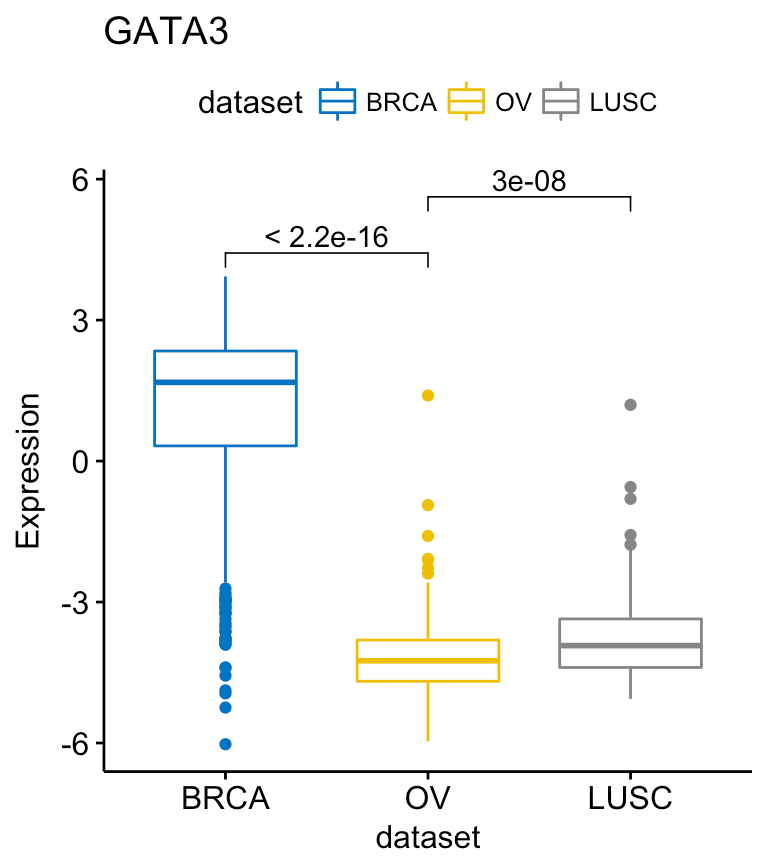Exploratory Data visualization: Gene Expression Data

For each of the genes, you can compare the different groups as follow:

`compare_means(c(GATA3, PTEN, XBP1) ~ dataset, data = expr)`

```# A tibble: 9 x 8
.y. group1 group2             p         p.adj p.format p.signif   method
<fctr>  <chr>  <chr>         <dbl>         <dbl>    <chr>    <chr>    <chr>
1  GATA3   BRCA     OV 1.111768e-177 3.335304e-177  < 2e-16     **** Wilcoxon
2  GATA3   BRCA   LUSC  6.684016e-73  1.336803e-72  < 2e-16     **** Wilcoxon
3  GATA3     OV   LUSC  2.965702e-08  2.965702e-08  3.0e-08     **** Wilcoxon
4   PTEN   BRCA     OV  6.791940e-05  6.791940e-05  6.8e-05     **** Wilcoxon
5   PTEN   BRCA   LUSC  1.042830e-16  3.128489e-16  < 2e-16     **** Wilcoxon
6   PTEN     OV   LUSC  1.280576e-07  2.561153e-07  1.3e-07     **** Wilcoxon
7   XBP1   BRCA     OV 2.551228e-123 7.653685e-123  < 2e-16     **** Wilcoxon
8   XBP1   BRCA   LUSC  1.950162e-42  3.900324e-42  < 2e-16     **** Wilcoxon
9   XBP1     OV   LUSC  4.239570e-11  4.239570e-11  4.2e-11     **** Wilcoxon```

If you want to select items (here cancer types) to display or to remove a particular item from the plot, use the argument select or remove, as follow:

```# Select BRCA and OV cancer types
ggboxplot(expr, x = "dataset", y = "GATA3",
title = "GATA3", ylab = "Expression",
color = "dataset", palette = "jco",
select = c("BRCA", "OV"))

# or remove BRCA
ggboxplot(expr, x = "dataset", y = "GATA3",
title = "GATA3", ylab = "Expression",
color = "dataset", palette = "jco",
remove = "BRCA")```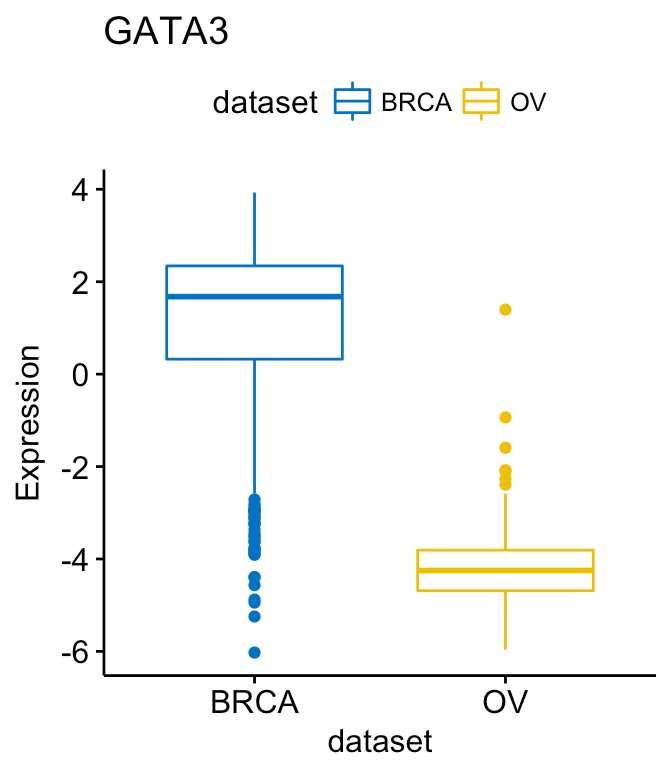Exploratory Data visualization: Gene Expression Data

To change the order of the data sets on x axis, use the argument order. For example order = c(“LUSC”, “OV”, “BRCA”):

```# Order data sets
ggboxplot(expr, x = "dataset", y = "GATA3",
title = "GATA3", ylab = "Expression",
color = "dataset", palette = "jco",
order = c("LUSC", "OV", "BRCA"))```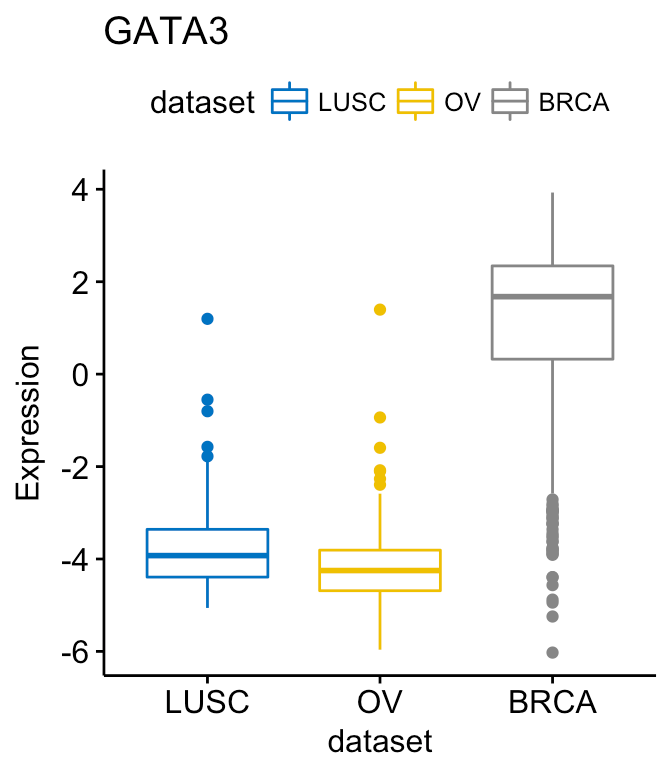Exploratory Data visualization: Gene Expression Data

To create horizontal plots, use the argument rotate = TRUE:

```ggboxplot(expr, x = "dataset", y = "GATA3",
title = "GATA3", ylab = "Expression",
color = "dataset", palette = "jco",
rotate = TRUE)```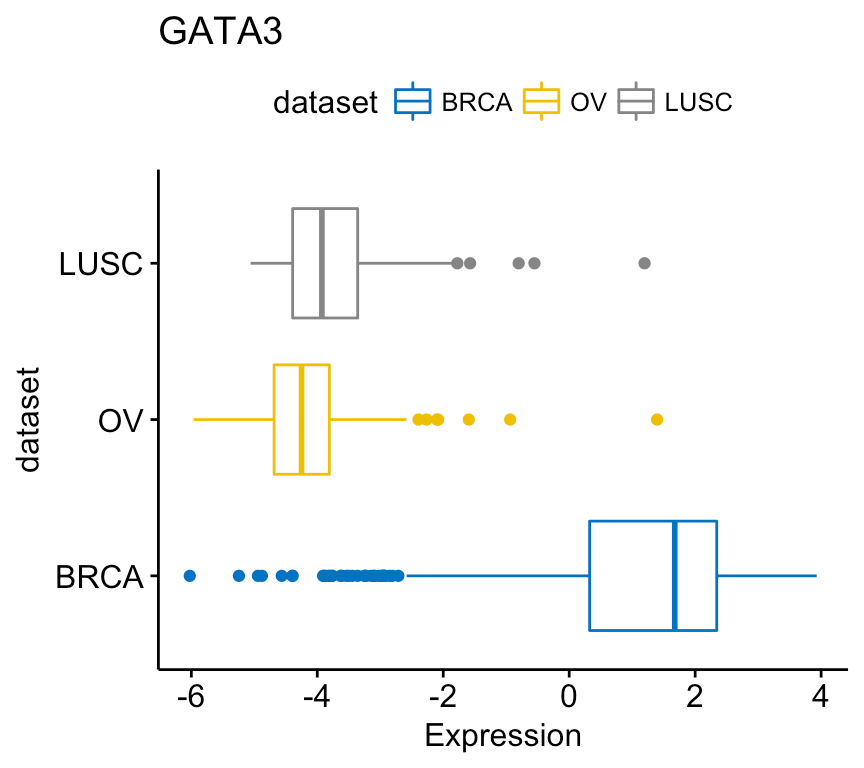Exploratory Data visualization: Gene Expression Data

To combine the three gene expression plots into a multi-panel plot, use the argument combine = TRUE:

```ggboxplot(expr, x = "dataset",
y = c("GATA3", "PTEN", "XBP1"),
combine = TRUE,
ylab = "Expression",
color = "dataset", palette = "jco")```Exploratory Data visualization: Gene Expression Data

You can also merge the 3 plots using the argument merge = TRUE or merge = “asis”:

```ggboxplot(expr, x = "dataset",
y = c("GATA3", "PTEN", "XBP1"),
merge = TRUE,
ylab = "Expression",
palette = "jco")```Exploratory Data visualization: Gene Expression Data

In the plot above, It’s easy to visually compare the expression level of the different genes in each cancer type.

But you might want to put genes (y variables) on x axis, in order to compare the expression level in the different cell subpopulations.

In this situation, the y variables (i.e.: genes) become x tick labels and the x variable (i.e.: dataset) becomes the grouping variable. To do this, use the argument merge = “flip”.

```ggboxplot(expr, x = "dataset",
y = c("GATA3", "PTEN", "XBP1"),
merge = "flip",
ylab = "Expression",
palette = "jco")```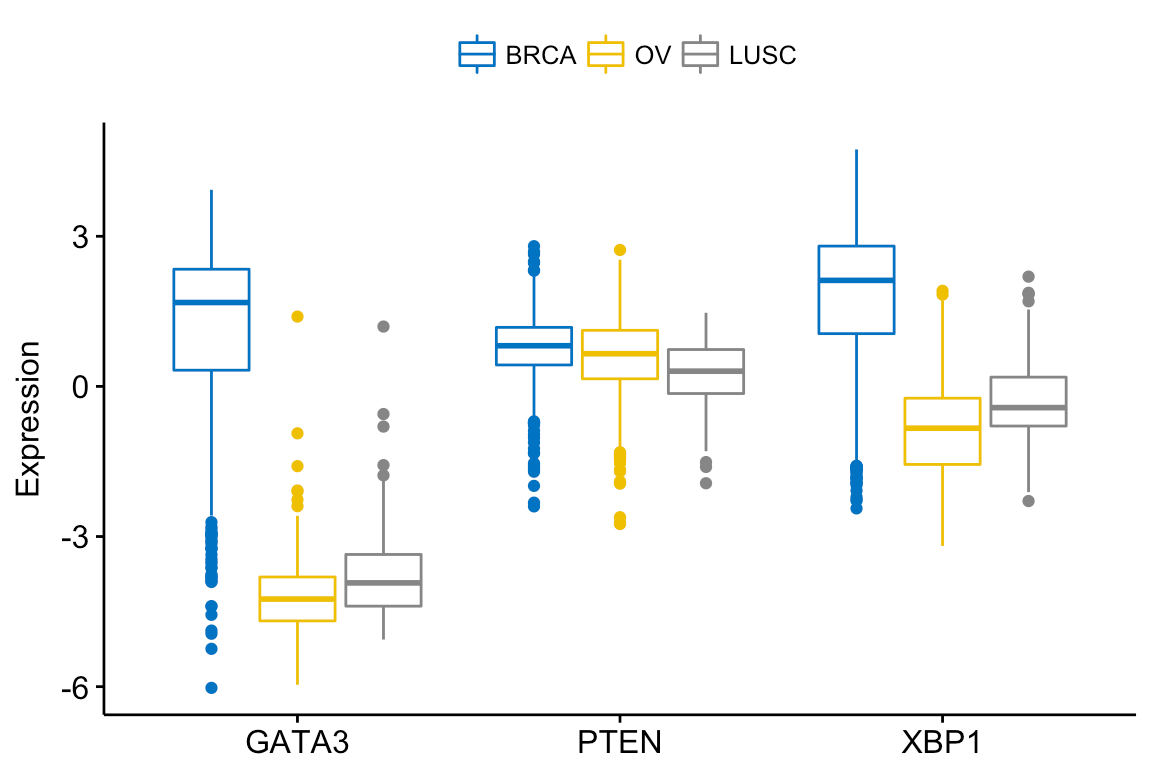Exploratory Data visualization: Gene Expression Data

You might want to add jittered points on the boxplot. Each point correspond to individual observations. To add jittered points, use the argument add = “jitter” as follow. To customize the added elements, specify the argument add.params.

```ggboxplot(expr, x = "dataset",
y = c("GATA3", "PTEN", "XBP1"),
combine = TRUE,
color = "dataset", palette = "jco",
ylab = "Expression",
add.params = list(size = 0.1, jitter = 0.2)  # Point size and the amount of jittering
)```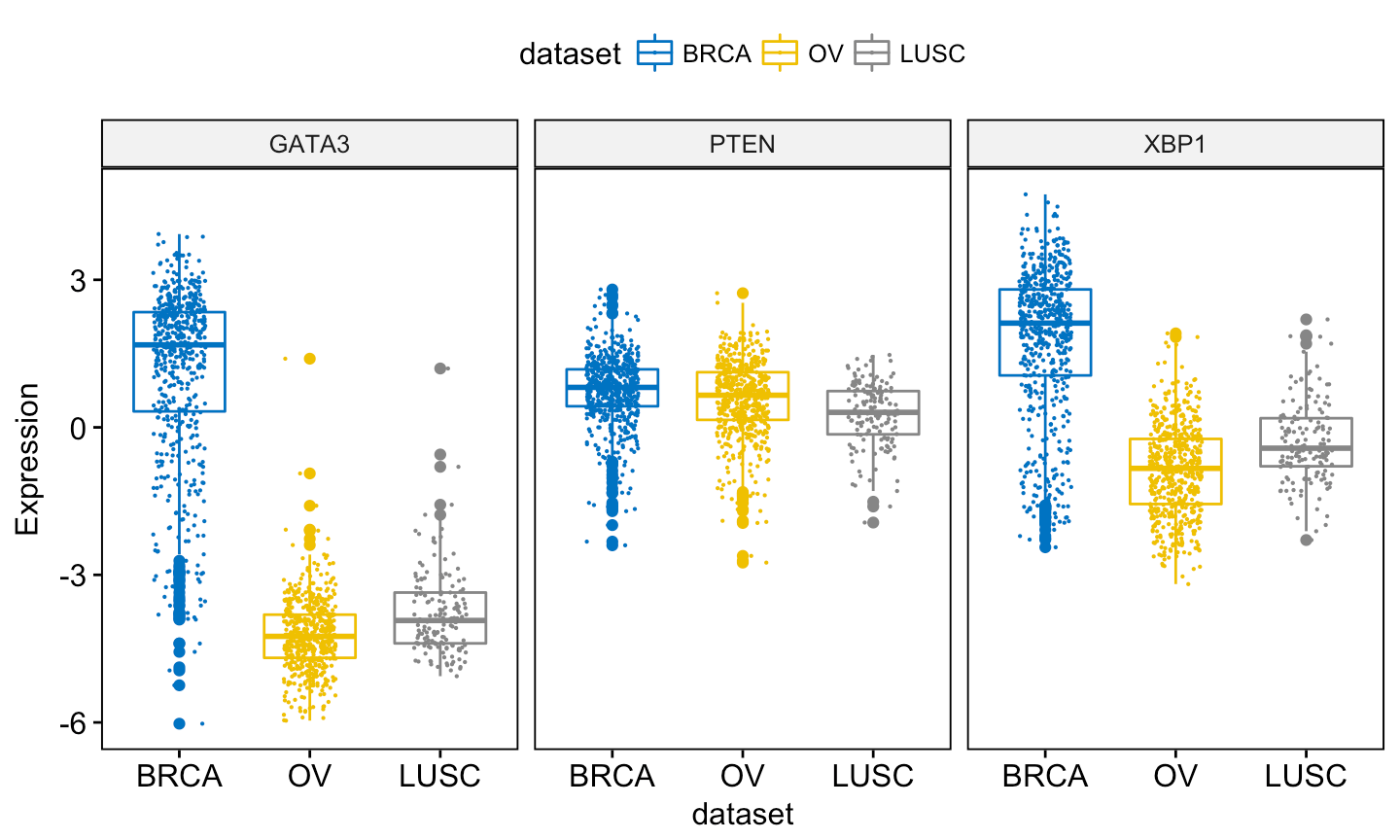Exploratory Data visualization: Gene Expression Data

Note that, when using ggboxplot() sensible values for the argument add are one of c(“jitter”, “dotplot”). If you decide to use add = “dotplot”, you can adjust dotsize and binwidth wen you have a strong dense dotplot. Read more about binwidth.

```ggboxplot(expr, x = "dataset",
y = c("GATA3", "PTEN", "XBP1"),
combine = TRUE,
color = "dataset", palette = "jco",
ylab = "Expression",
add.params = list(binwidth = 0.1, dotsize = 0.3)
)```Exploratory Data visualization: Gene Expression Data

You might want to label the boxplot by showing the names of samples with the top n highest or lowest values. In this case, you can use the following arguments:

• label: the name of the column containing point labels.
• label.select: can be of two formats:
• a character vector specifying some labels to show.
• a list containing one or the combination of the following components:
• top.up and top.down: to display the labels of the top up/down points. For example, label.select = list(top.up = 10, top.down = 4).
• criteria: to filter, for example, by x and y variables values, use this: label.select = list(criteria = “`y` > 3.9 & `y` < 5 & `x` %in% c(‘BRCA’, ‘OV’)”).

For example:

```ggboxplot(expr, x = "dataset",
y = c("GATA3", "PTEN", "XBP1"),
combine = TRUE,
color = "dataset", palette = "jco",
ylab = "Expression",
add.params = list(size = 0.1, jitter = 0.2),  # Point size and the amount of jittering
label = "bcr_patient_barcode",                # column containing point labels
label.select = list(top.up = 2, top.down = 2),# Select some labels to display
font.label = list(size = 9, face = "italic"), # label font
repel = TRUE                                  # Avoid label text overplotting
)```Exploratory Data visualization: Gene Expression Data

A complex criteria for labeling can be specified as follow:

```label.select.criteria <- list(criteria = "`y` > 3.9 & `x` %in% c('BRCA', 'OV')")
ggboxplot(expr, x = "dataset",
y = c("GATA3", "PTEN", "XBP1"),
combine = TRUE,
color = "dataset", palette = "jco",
ylab = "Expression",
label = "bcr_patient_barcode",              # column containing point labels
label.select = label.select.criteria,       # Select some labels to display
font.label = list(size = 9, face = "italic"), # label font
repel = TRUE                                # Avoid label text overplotting
)```

Other types of plots, with the same arguments as the function ggboxplot(), are available, such as stripchart and violin plots.

## Violin plots

The following R code draws violin plots with box plots inside:

```ggviolin(expr, x = "dataset",
y = c("GATA3", "PTEN", "XBP1"),
combine = TRUE,
color = "dataset", palette = "jco",
ylab = "Expression",Exploratory Data visualization: Gene Expression Data

Instead of adding a box plot inside the violin plot, you can add the median + interquantile range as follow:

```ggviolin(expr, x = "dataset",
y = c("GATA3", "PTEN", "XBP1"),
combine = TRUE,
color = "dataset", palette = "jco",
ylab = "Expression",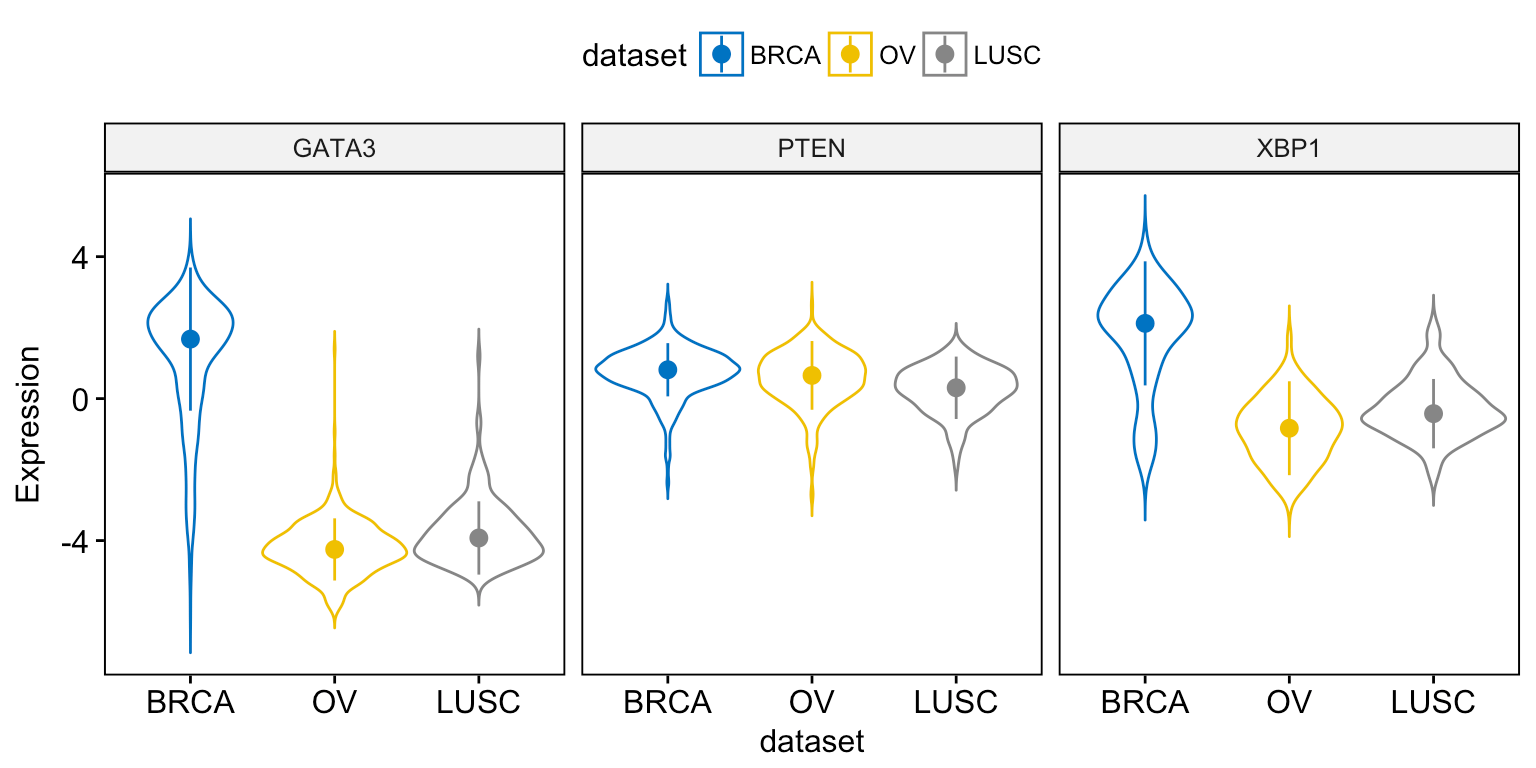Exploratory Data visualization: Gene Expression Data

When using the function ggviolin(), sensible values for the argument add include: “mean”, “mean_se”, “mean_sd”, “mean_ci”, “mean_range”, “median”, “median_iqr”, “median_mad”, “median_range”.

You can also add “jitter” points and “dotplot” inside the violin plot as described previously in the box plot section.

## Stripcharts and dot plots

To draw a stripchart, type this:

```ggstripchart(expr, x = "dataset",
y = c("GATA3", "PTEN", "XBP1"),
combine = TRUE,
color = "dataset", palette = "jco",
size = 0.1, jitter = 0.2,
ylab = "Expression",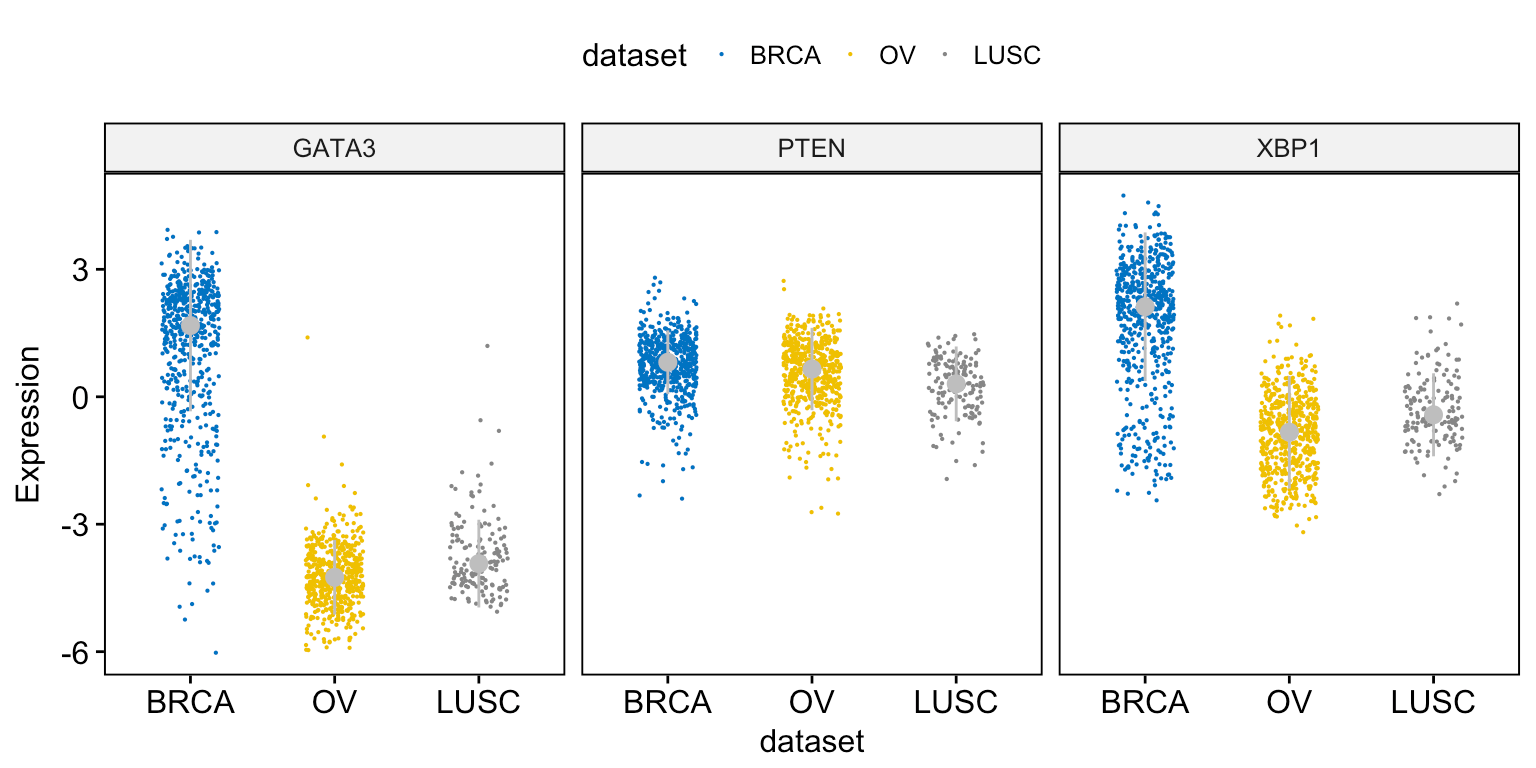Exploratory Data visualization: Gene Expression Data

For a dot plot, use this:

```ggdotplot(expr, x = "dataset",
y = c("GATA3", "PTEN", "XBP1"),
combine = TRUE,
color = "dataset", palette = "jco",
fill = "white",
binwidth = 0.1,
ylab = "Expression",Exploratory Data visualization: Gene Expression Data

## Density plots

To visualize the distribution as a density plot, use the function ggdensity() as follow:

```# Basic density plot
ggdensity(expr,
x = c("GATA3", "PTEN",  "XBP1"),
y = "..density..",
combine = TRUE,                  # Combine the 3 plots
xlab = "Expression",
rug = TRUE                       # Add marginal rug
)```Exploratory Data visualization: Gene Expression Data

```# Change color and fill by dataset
ggdensity(expr,
x = c("GATA3", "PTEN",  "XBP1"),
y = "..density..",
combine = TRUE,                  # Combine the 3 plots
xlab = "Expression",
rug = TRUE,                      # Add marginal rug
color = "dataset",
fill = "dataset",
palette = "jco"
)```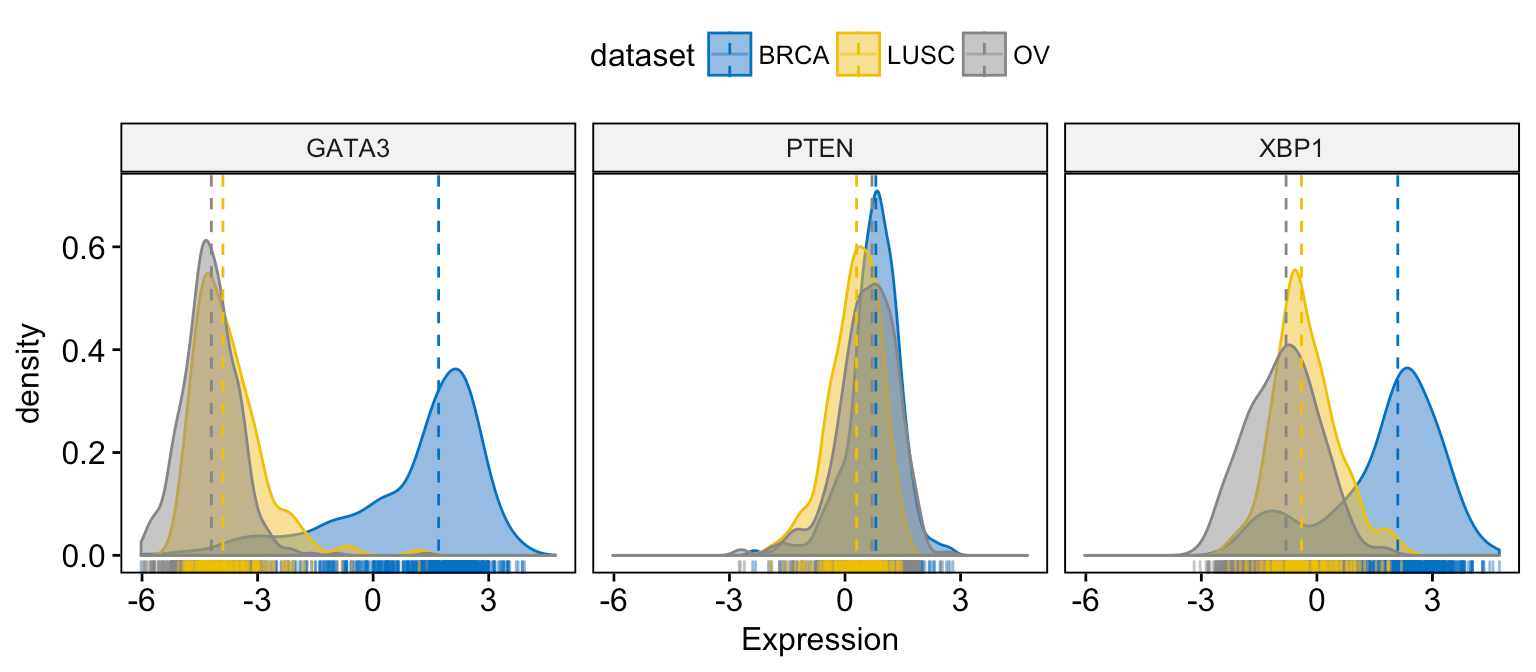Exploratory Data visualization: Gene Expression Data

```# Merge the 3 plots
# and use y = "..count.." instead of "..density.."
ggdensity(expr,
x = c("GATA3", "PTEN",  "XBP1"),
y = "..count..",
merge = TRUE,                    # Merge the 3 plots
xlab = "Expression",
rug = TRUE ,                     # Add marginal rug
palette = "jco"                  # Change color palette
)```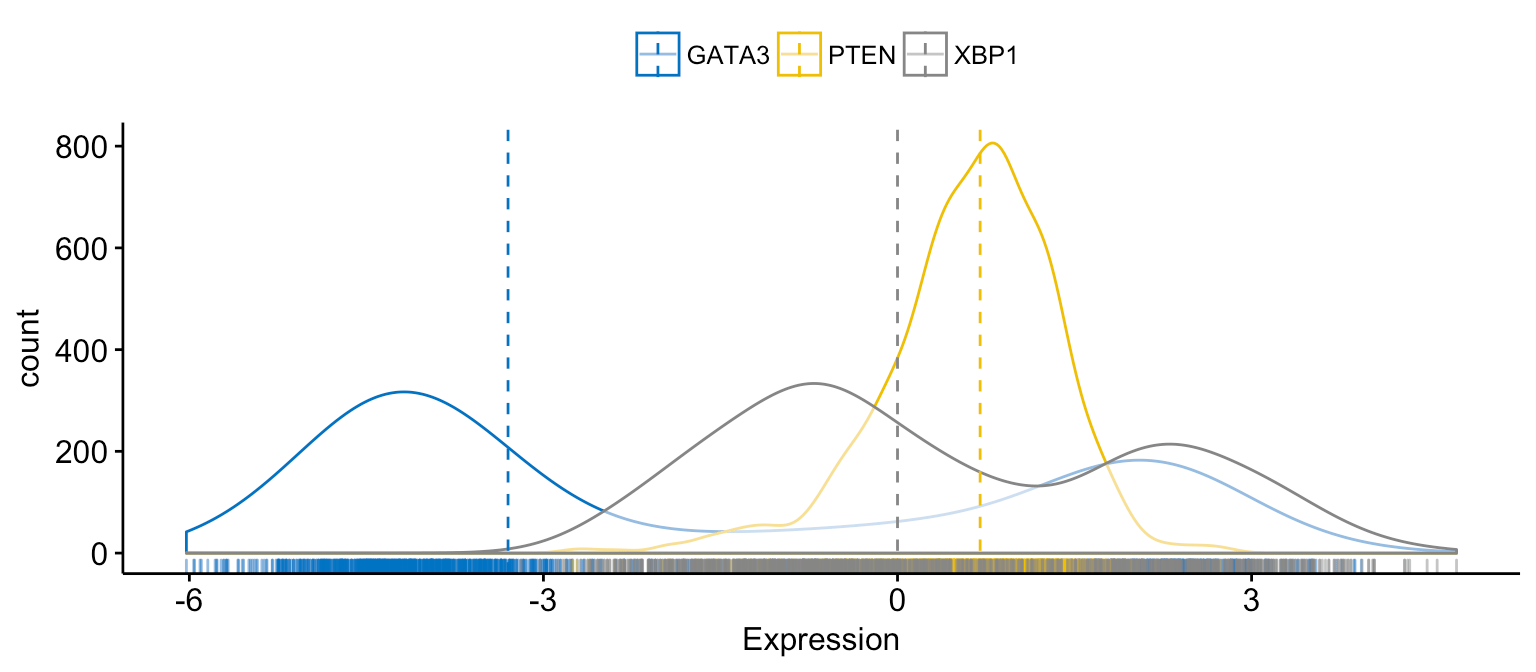Exploratory Data visualization: Gene Expression Data

```# color and fill by x variables
ggdensity(expr,
x = c("GATA3", "PTEN",  "XBP1"),
y = "..count..",
color = ".x.", fill = ".x.",     # color and fill by x variables
merge = TRUE,                    # Merge the 3 plots
xlab = "Expression",
rug = TRUE ,                     # Add marginal rug
palette = "jco"                  # Change color palette
)```Exploratory Data visualization: Gene Expression Data

```# Facet by "dataset"
ggdensity(expr,
x = c("GATA3", "PTEN",  "XBP1"),
y = "..count..",
color = ".x.", fill = ".x.",
facet.by = "dataset",            # Split by "dataset" into multi-panel
merge = TRUE,                    # Merge the 3 plots
xlab = "Expression",
rug = TRUE ,                     # Add marginal rug
palette = "jco"                  # Change color palette
)```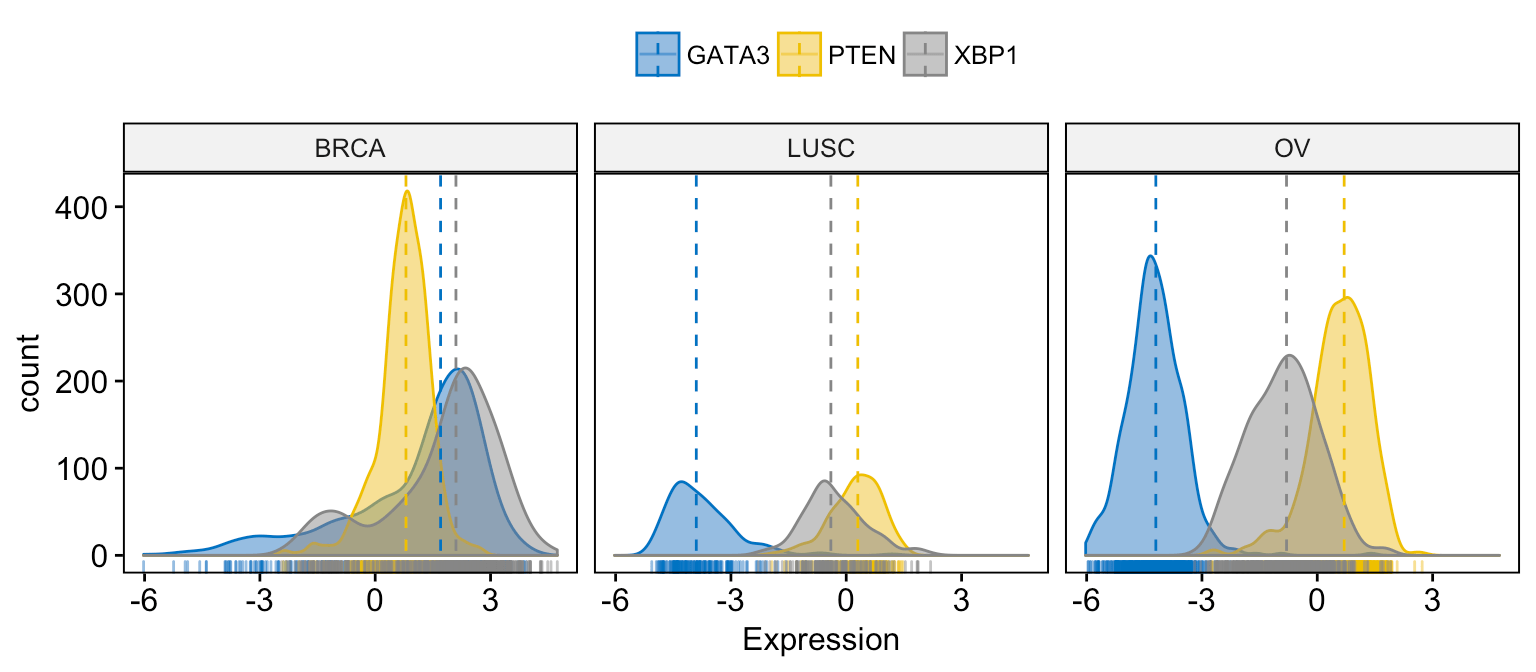Exploratory Data visualization: Gene Expression Data

## Histogram plots

To visualize the distribution as a histogram plot, use the function gghistogram() as follow:

```# Basic histogram plot
gghistogram(expr,
x = c("GATA3", "PTEN",  "XBP1"),
y = "..density..",
combine = TRUE,                  # Combine the 3 plots
xlab = "Expression",
rug = TRUE                       # Add marginal rug
)```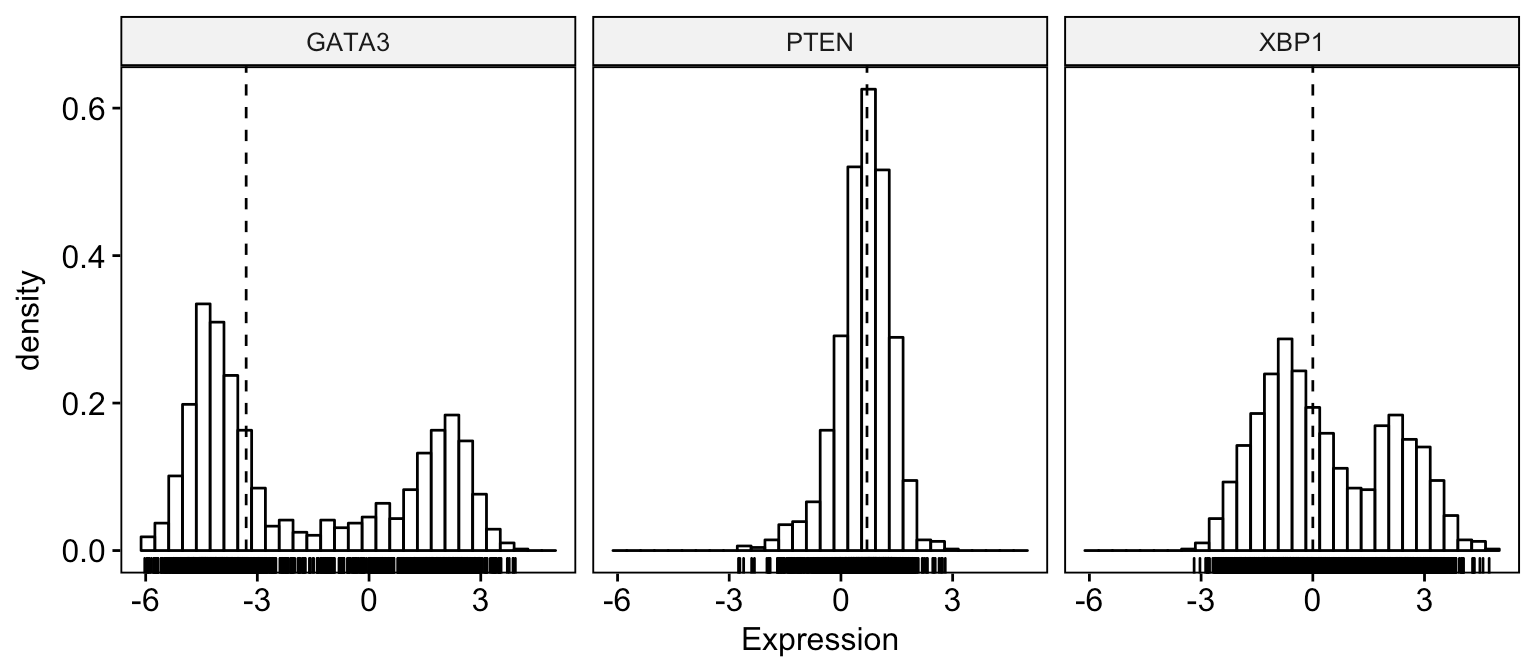Exploratory Data visualization: Gene Expression Data

```# Change color and fill by dataset
gghistogram(expr,
x = c("GATA3", "PTEN",  "XBP1"),
y = "..density..",
combine = TRUE,                  # Combine the 3 plots
xlab = "Expression",
rug = TRUE,                      # Add marginal rug
color = "dataset",
fill = "dataset",
palette = "jco"
)```Exploratory Data visualization: Gene Expression Data

```# Merge the 3 plots
# and use y = "..count.." instead of "..density.."
gghistogram(expr,
x = c("GATA3", "PTEN",  "XBP1"),
y = "..count..",
merge = TRUE,                    # Merge the 3 plots
xlab = "Expression",
rug = TRUE ,                     # Add marginal rug
palette = "jco"                  # Change color palette
)```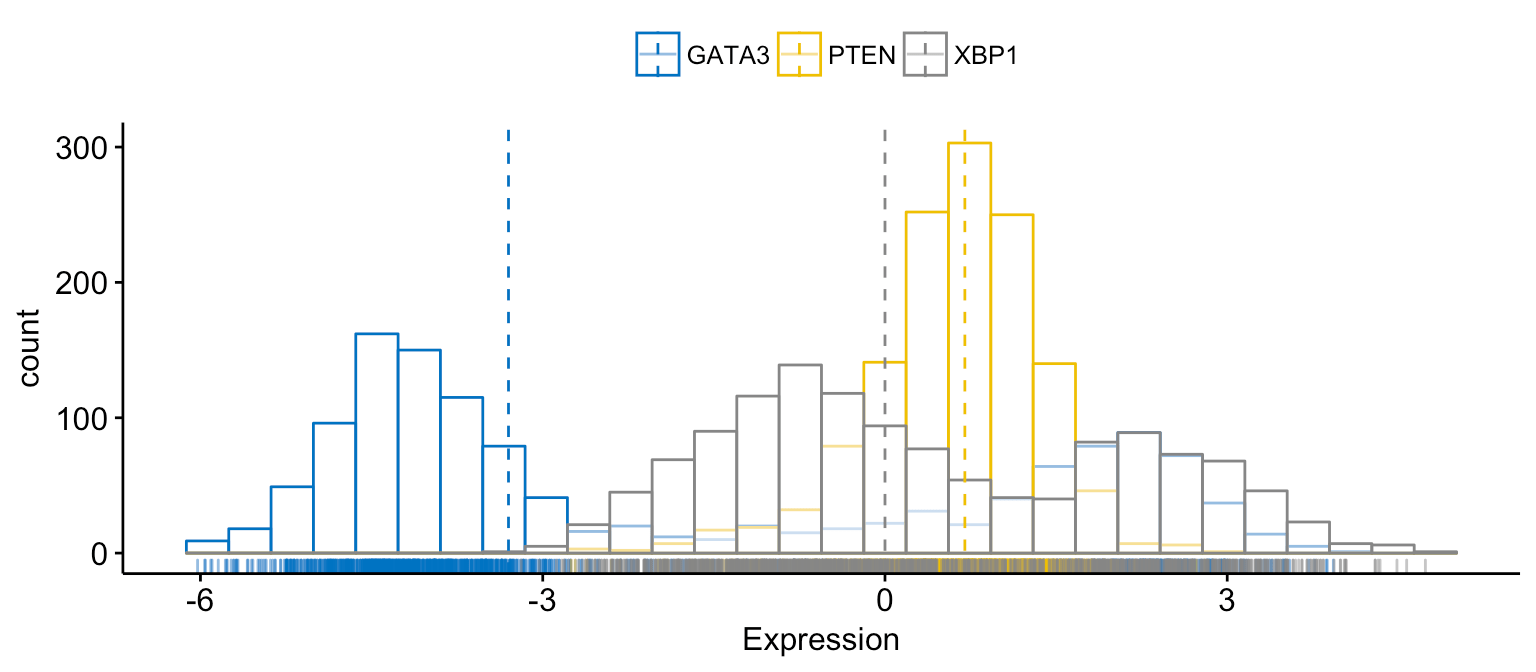Exploratory Data visualization: Gene Expression Data

```# color and fill by x variables
gghistogram(expr,
x = c("GATA3", "PTEN",  "XBP1"),
y = "..count..",
color = ".x.", fill = ".x.",     # color and fill by x variables
merge = TRUE,                    # Merge the 3 plots
xlab = "Expression",
rug = TRUE ,                     # Add marginal rug
palette = "jco"                  # Change color palette
)```Exploratory Data visualization: Gene Expression Data

```# Facet by "dataset"
gghistogram(expr,
x = c("GATA3", "PTEN",  "XBP1"),
y = "..count..",
color = ".x.", fill = ".x.",
facet.by = "dataset",            # Split by "dataset" into multi-panel
merge = TRUE,                    # Merge the 3 plots
xlab = "Expression",
rug = TRUE ,                     # Add marginal rug
palette = "jco"                  # Change color palette
)```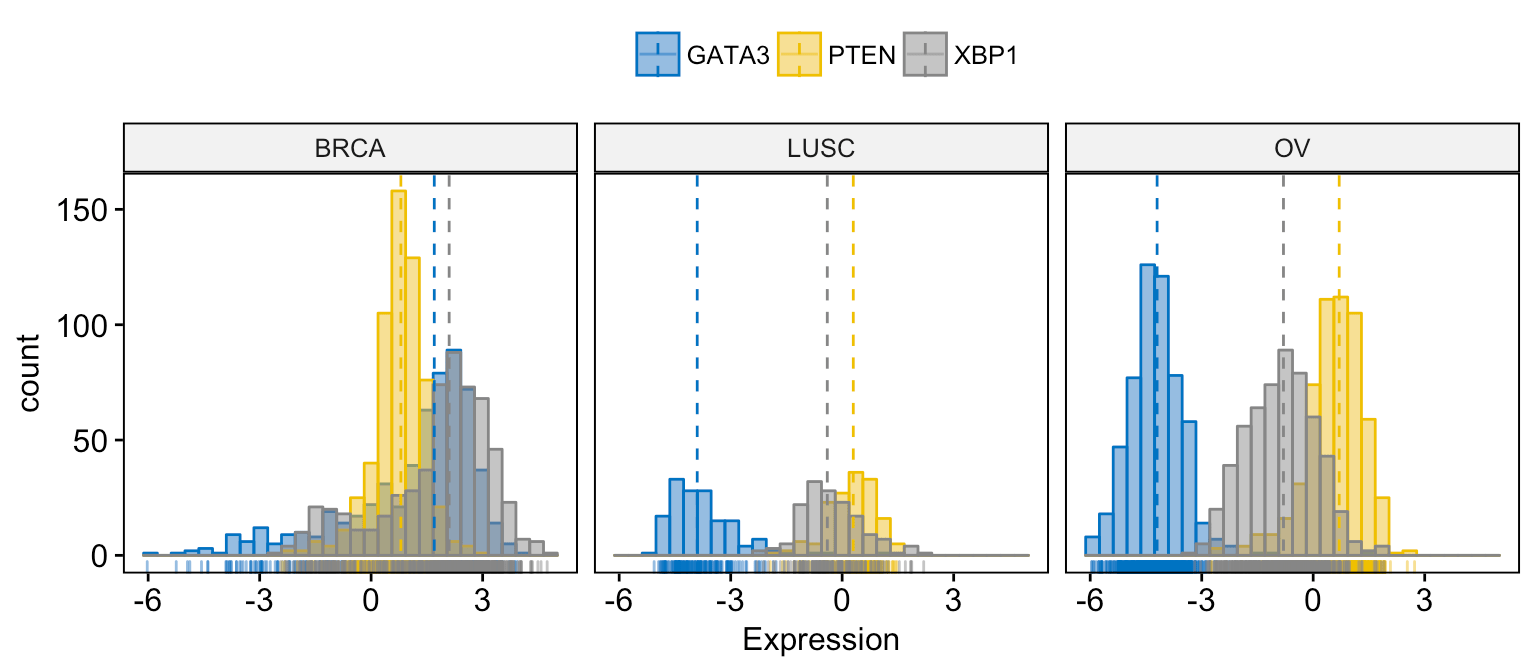Exploratory Data visualization: Gene Expression Data

## Empirical cumulative density function

```# Basic ECDF plot
ggecdf(expr,
x = c("GATA3", "PTEN",  "XBP1"),
combine = TRUE,
xlab = "Expression", ylab = "F(expression)"
)```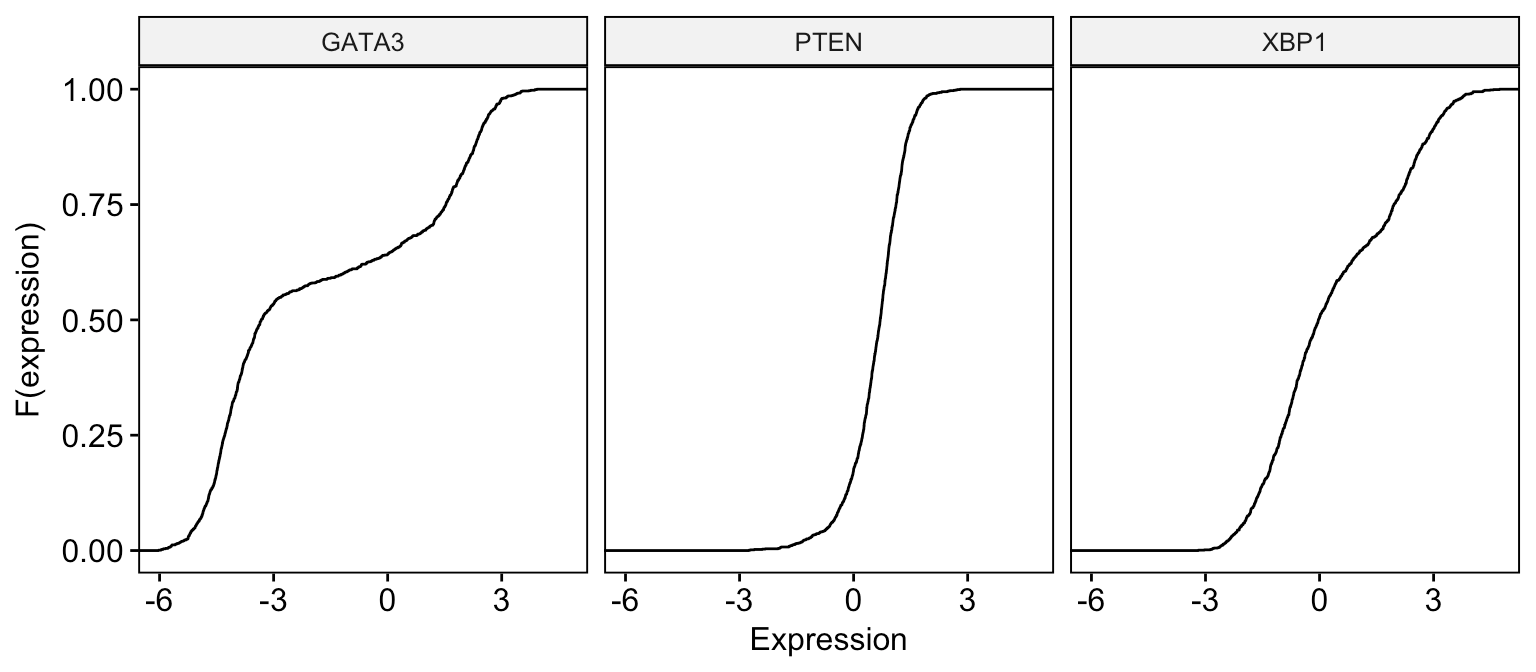Exploratory Data visualization: Gene Expression Data

```# Change color  by dataset
ggecdf(expr,
x = c("GATA3", "PTEN",  "XBP1"),
combine = TRUE,
xlab = "Expression", ylab = "F(expression)",
color = "dataset", palette = "jco"
)```Exploratory Data visualization: Gene Expression Data

```# Merge the 3 plots and color by x variables
ggecdf(expr,
x = c("GATA3", "PTEN",  "XBP1"),
merge = TRUE,
xlab = "Expression", ylab = "F(expression)",
color = ".x.", palette = "jco"
)```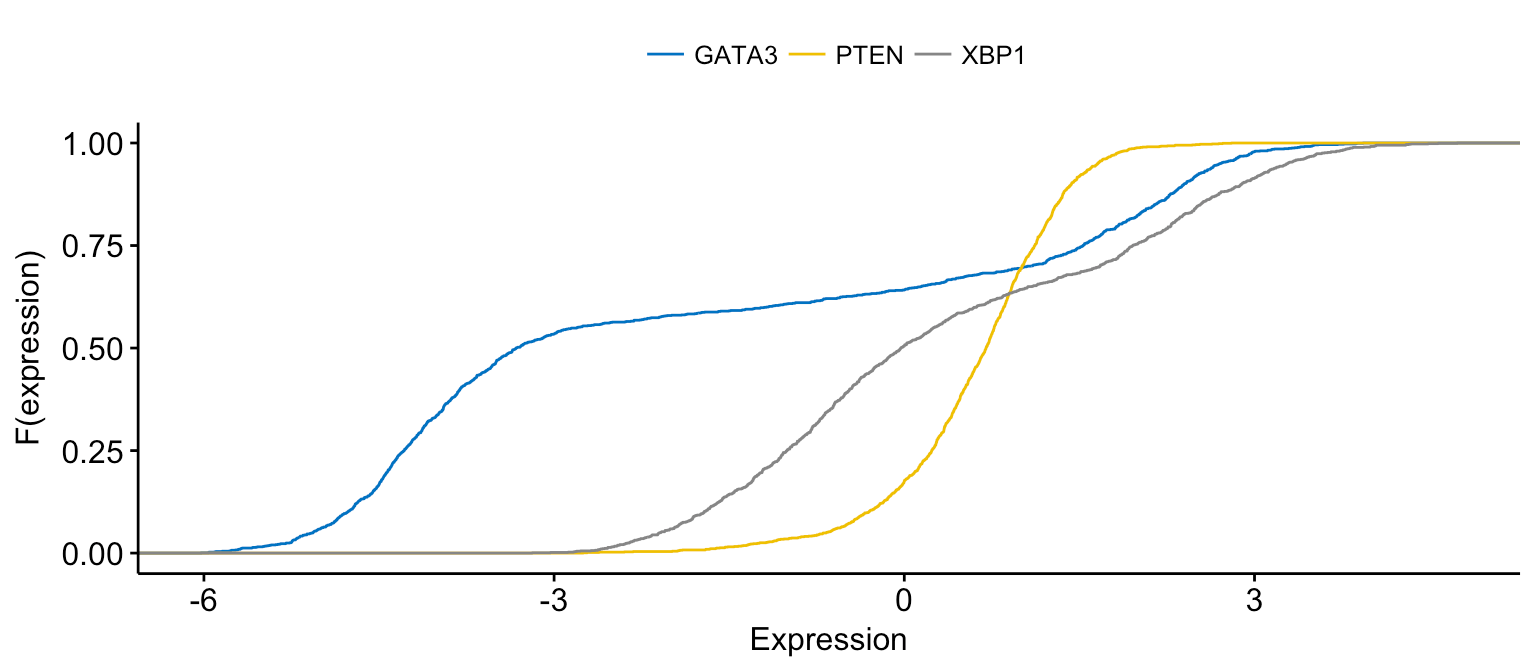Exploratory Data visualization: Gene Expression Data

```# Merge the 3 plots and color by x variables
# facet by "dataset" into multi-panel
ggecdf(expr,
x = c("GATA3", "PTEN",  "XBP1"),
merge = TRUE,
xlab = "Expression", ylab = "F(expression)",
color = ".x.", palette = "jco",
facet.by = "dataset"
)```Exploratory Data visualization: Gene Expression Data

## Quantile – Quantile plot

```# Basic ECDF plot
ggqqplot(expr,
x = c("GATA3", "PTEN",  "XBP1"),
combine = TRUE, size = 0.5
)```Exploratory Data visualization: Gene Expression Data

```# Change color  by dataset
ggqqplot(expr,
x = c("GATA3", "PTEN",  "XBP1"),
combine = TRUE, color = "dataset", palette = "jco",
size = 0.5
)```Exploratory Data visualization: Gene Expression Data

```# Merge the 3 plots and color by x variables
ggqqplot(expr,
x = c("GATA3", "PTEN",  "XBP1"),
merge = TRUE,
color = ".x.", palette = "jco"
)```Exploratory Data visualization: Gene Expression Data

```# Merge the 3 plots and color by x variables
# facet by "dataset" into multi-panel
ggqqplot(expr,
x = c("GATA3", "PTEN",  "XBP1"),
merge = TRUE, size = 0.5,
color = ".x.", palette = "jco",
facet.by = "dataset"
)```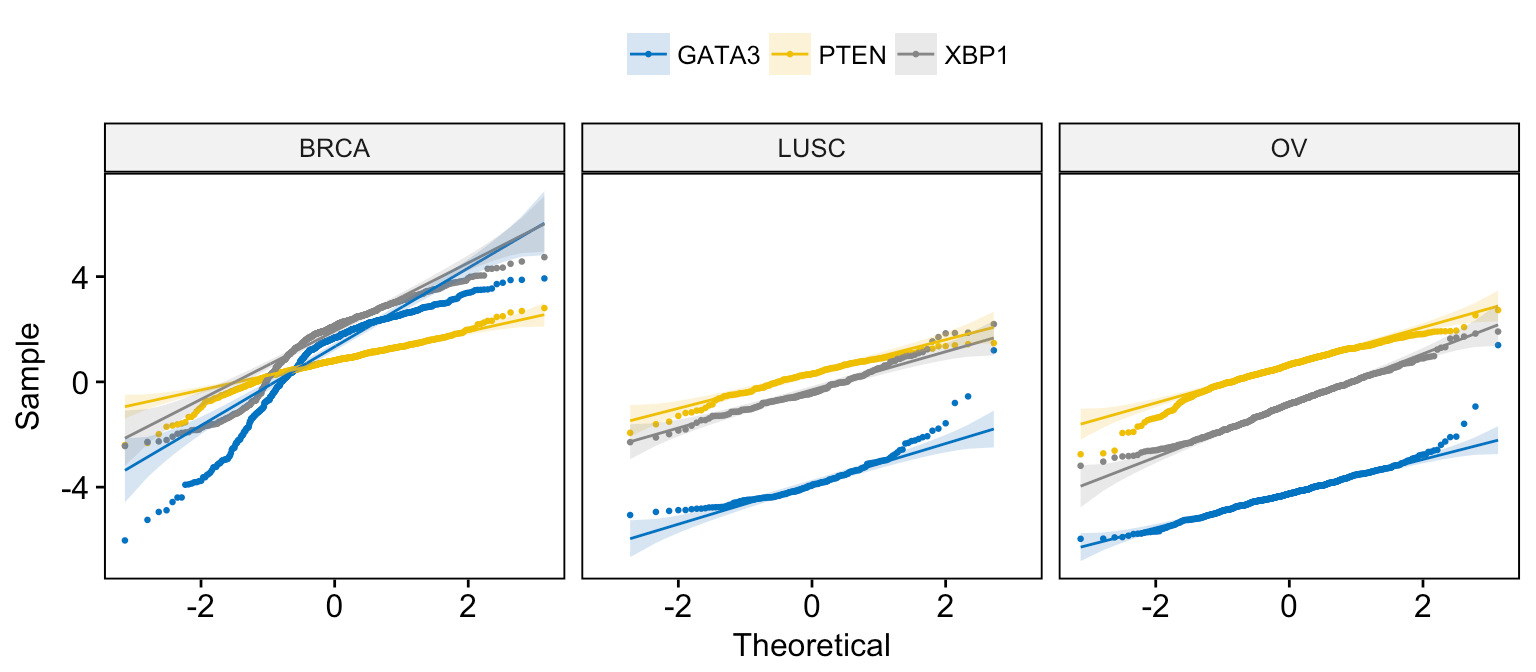Exploratory Data visualization: Gene Expression Data

## Infos

This analysis has been performed using R software (ver. 3.3.2) and ggpubr (ver. 0.1.3).